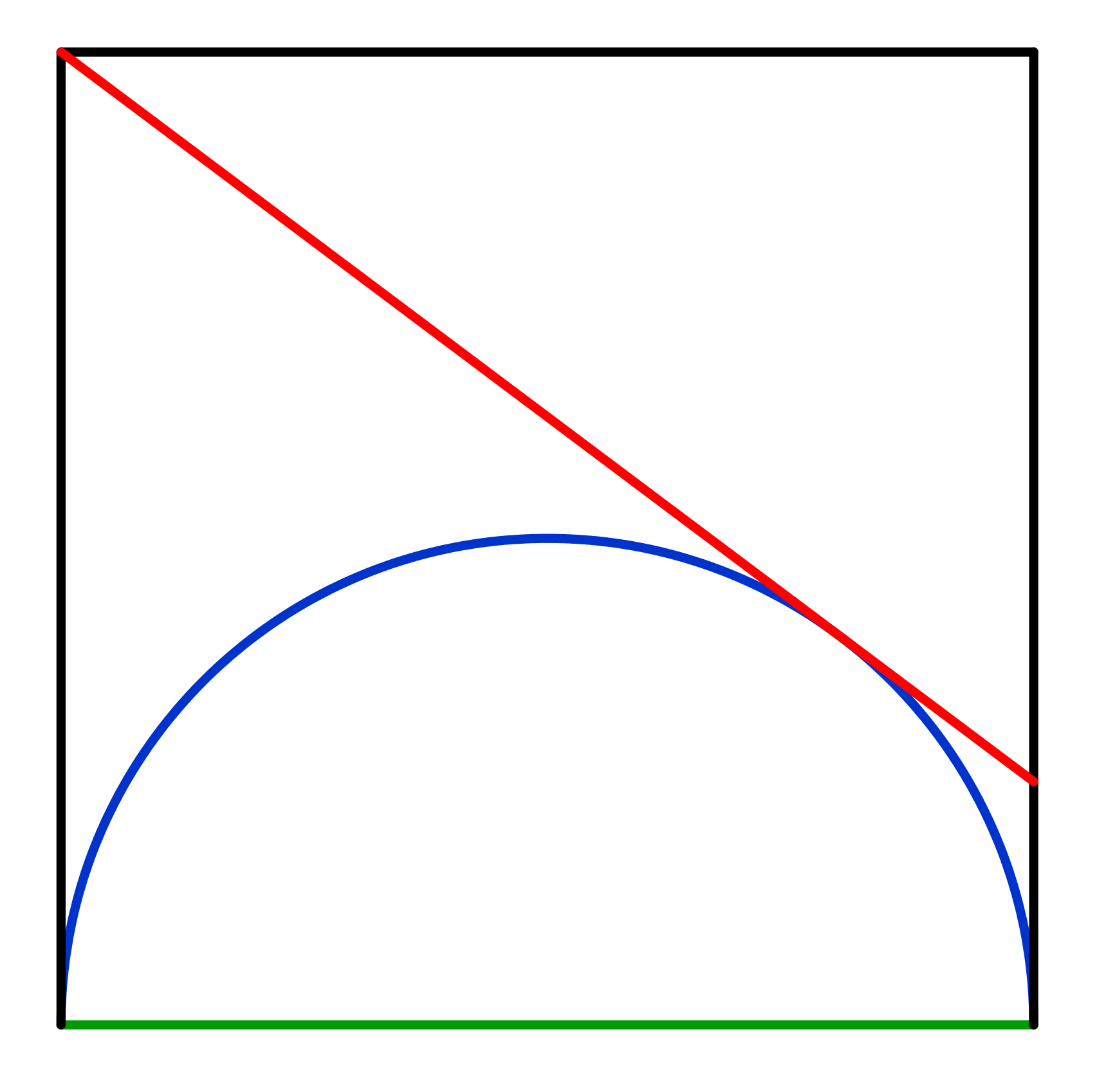# Rojo, azul y verde

Geometry Level 2The figure above shows a square with perimeter 32 cm. The diameter of the inscribed blue semicircle is the green side of the square. A red tangent to the blue semicircle is drawn joining the square upper left vertex and a point on its right side. Find the length of the red tangent in cm.

×